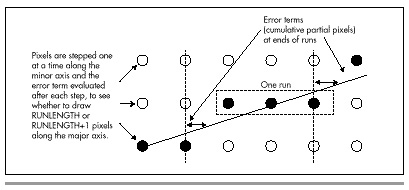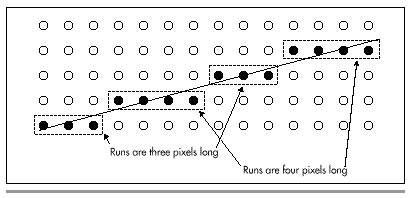The run-length slice algorithm rotates matters 90 degrees, with salubrious results. The basis of the run-length slice algorithm is stepping one pixel at a time along the minor axis (the shorter dimension), while maintaining an integer error term indicating how close the line is to advancing an extra pixel along the major axis, as illustrated by Figure 36.2.

Consider this: When you’re called upon to draw a line with an X-dimension of 35 and a Y-dimension of 10, you have a great deal of information available, some of which is ignored by standard Bresenham’s. In particular, because the slope is between 1/3 and 1/4, you know that every single run—a run being a set of pixels at the same minor-axis coordinate—must be either three or four pixels long. No other length is possible, as shown in Figure 36.3 (apart from the first and last runs, which are special cases that I’ll discuss shortly). Therefore, for this line, there’s no need to perform an error-term calculation and test for each pixel. Instead, we can just perform one test per run, to see whether the run is three or four pixels long, thereby eliminating about 70 percent of the calculations in drawing this line.

Take a moment to let the idea behind run-length slice drawing soak in. Periodic decisions must be made to control pixel placement. The key to speed is to make those decisions as infrequently and as quickly as possible. Of course, it will work to make a decision at each pixel—that’s standard Bresenham’s. However, most of those per-pixel decisions are redundant, and in fact we have enough information before we begin drawing to know which are the redundant decisions. Run-length slice drawing is exactly equivalent to standard Bresenham’s, but it pares the decision-making process down to a minimum. It’s somewhat analogous to the difference between finding the greatest common divisor of two numbers using Euclid’s algorithm and finding it by trying every possible divisor. Both approaches produce the desired result, but that which takes maximum advantage of the available information and minimizes redundant work is preferable.Figure 36.2
Run-length slice line drawing.Figure 36.3
Runs in a slope 1/3.5 line.

### Run-Length Slice Implementation

We know that for any line, a given run will always be one of two possible lengths. How, though, do we know which length to select? Surprisingly, this is easy to determine. For the following discussion, assume that we have a slope of 1/3.5, so that X is the major axis; however, the discussion also applies to Y-major lines, with X and Y reversed.

The minimum possible length for any run in an X-major line is int(XDelta/YDelta), where XDelta is the X-dimension of the line and YDelta is the Y-dimension. The maximum possible length is int(XDelta/YDelta)+ 1. The trick, then, is knowing which of these two lengths to select for each run. To see how we can make this selection, refer to Figure 36.4. For each one-pixel step along the minor axis (Y, in this case), we advance at least three pixels. The full advance distance along X (the major axis) is actually three-plus pixels, because there is also a fractional portion to the advance along X for a single-pixel Y step. This fractional advance is the key to deciding when to add an extra pixel to a run. The fraction indicates what portion of an extra pixel we advance along X (the major axis) during each run. If we keep a running sum of the fractional parts, we have a measure of how close we are to needing an extra pixel; when the fractional sum reaches 1, it’s time to add an extra pixel to the current run. Then, we can subtract 1 from the running sum (because we just advanced one pixel), and continue on.Figure 36.4
How the error term determines run length.

Practically speaking, however, we can’t work with fractions because floating-point arithmetic is slow and fixed-point arithmetic is imprecise. Therefore, we take a cue from standard Bresenham’s and scale all the error-term calculations up so that we can work with integers. The fractional X (major axis) advance per one-pixel Y (minor axis) advance is the fractional portion of XDelta/YDelta. This value is exactly equivalent to (XDelta % YDelta)/YDelta. We’ll scale this up by multiplying it by YDelta*2, so that the amount by which we adjust the error term up for each one-pixel minor-axis advance is (XDelta % YDelta)*2.

We’ll similarly scale up the one pixel by which we adjust the error term down after it turns over, so our downward error-term adjustment is YDelta*2. Therefore, before drawing each run, we’ll add (XDelta % YDelta)*2 to the error term. If the error term runs over (reaches one full pixel), we’ll lengthen the run by 1, and subtract YDelta*2 from the error term. (All values are multiplied by 2 so that the initial error term, which involves a 0.5 term, can be scaled up to an integer, as discussed next.)

This is not a complicated process; it involves only integer addition and subtraction and a single test, and it lends itself to many and varied optimizations. For example, you could break out hardwired optimizations for drawing each possible pair of run lengths. For the aforementioned line with a slope of 1/3.5, for example, you could have one routine hardwired to blast in a run of three pixels as quickly as possible, and another hardwired to blast in a run of four pixels. These routines would ideally have no looping, but rather just a series of instructions customized to draw the desired number of pixels at maximum speed. Each routine would know that the only possibilities for the length of the next run would be three and four, so they could increment the error term, then jump directly to the appropriate one of the two routines depending on whether the error term turned over. Properly implemented, it should be possible to reduce the average per-run overhead of line drawing to less than one branch, with only two additions and two tests (the number of runs must also be counted down), plus a subtraction half the time. On a 486, this amounts to something on the order of 150 nanoseconds of overhead per pixel, exclusive of the time required to actually write the pixel to display memory.

That’s good.

Graphics Programming Black Book © 2001 Michael Abrash